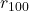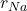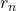## If the outermost electron in an atom is excited to a very high energy state, its orbit is far beyond that of the other electrons. To a good

Question

If the outermost electron in an atom is excited to a very high energy state, its orbit is far beyond that of the other electrons. To a good approximation, we can think of the electron as orbiting a compact core with a charge equal to the charge of a single proton. The outer electron in such a Rydberg atom thus has energy levels corresponding to those of hydrogen.
Sodium is a common element for such studies. How does the radius you calculated in part A compare to the approximately 0.20 nm radius of a typical sodium atom?
r100/rNa = _______.

in progress 0
6 months 2021-07-21T02:07:32+00:00 1 Answers 12 views 0

the calculated ratio to the radius of the sodium/is 2645.0

Explanation:

Given the data in the question;

the calculated ratio to the radius of the sodium =/so from here we can write the number of energy states as 100

The number of energy states; n = 100

A;

We know that the radius of the sodium atom is;= n²α₀

Now, the value of the Bohr radius; α₀ = 5.29 × 10⁻¹¹ m

so lets determine the radius of the sodium atom; by substituting in our values;= (100)² × (5.29 × 10⁻¹¹ m )= 5.29 × 10⁻⁷ m

B

given that, the theoretical value of the radius of the sodium is;= 0.2 nm = 2 × 10⁻¹⁰ m

so we calculate the ratio of the radii of the sodium;/= ( 5.29 × 10⁻⁷ m ) / ( 2 × 10⁻¹⁰ m )/= 2645.0

Therefore, the calculated ratio to the radius of the sodium/is 2645.0